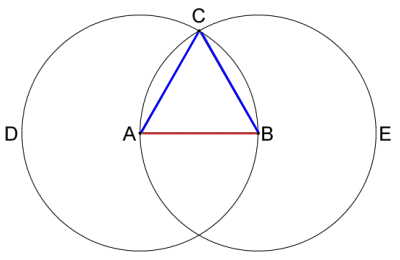# Straightedge and compass constructions

This is a quick note about straightedge and compass constructions, in relation to my set.

A quick definition: a straightedge is an arbitrarily long straight edge, and a compass is a circle drawing tool. Specifically, with a straight edge you can:

• Pick any two lines and draw the line through them

• Pick a point and draw a line through it

• Draw an arbitrary line

And with a compass you can:

• Pick two points, $A,B$ and draw a circle centered at $A$ with radius $|AB|$

• Pick a point and draw a circle centered at that point

• Draw an arbitrary circle going through a point

• Draw an arbitrary circle

Just a quick note about a compass, assume in the questions that you are using a non-collapsing compass, that is, if you draw a circle then you can make your next circle have the same radius as the circle you constructed free of charge.

I'll call a geometric construction constructible if it can be constructed with a straightedge and compass. For examplewhich shows that an equilateral triangle is constructible.

A constructible number, say $x$, is defined as follows:

If given a unit length, it is possible to construct a segment of length $x$.

Also a shape is constructible given another shape if it is possible to construct another shape given the first one. So although a $22$-gon is not constructible, it is constructible given an $11$-gon.

Lastly a use is a single use of a compass or straightedge, as defined above. So the equilateral triangle takes 5 moves.

Again this is in relation to my set.Note by Wen Z
4 years, 11 months ago

This discussion board is a place to discuss our Daily Challenges and the math and science related to those challenges. Explanations are more than just a solution — they should explain the steps and thinking strategies that you used to obtain the solution. Comments should further the discussion of math and science.

When posting on Brilliant:

• Use the emojis to react to an explanation, whether you're congratulating a job well done , or just really confused .
• Ask specific questions about the challenge or the steps in somebody's explanation. Well-posed questions can add a lot to the discussion, but posting "I don't understand!" doesn't help anyone.
• Try to contribute something new to the discussion, whether it is an extension, generalization or other idea related to the challenge.

MarkdownAppears as
*italics* or _italics_ italics
**bold** or __bold__ bold
- bulleted- list
• bulleted
• list
1. numbered2. list
1. numbered
2. list
Note: you must add a full line of space before and after lists for them to show up correctly
paragraph 1paragraph 2

paragraph 1

paragraph 2

[example link](https://brilliant.org)example link
> This is a quote
This is a quote
    # I indented these lines
# 4 spaces, and now they show
# up as a code block.

print "hello world"
# I indented these lines
# 4 spaces, and now they show
# up as a code block.

print "hello world"
MathAppears as
Remember to wrap math in $$ ... $$ or $ ... $ to ensure proper formatting.
2 \times 3 $2 \times 3$
2^{34} $2^{34}$
a_{i-1} $a_{i-1}$
\frac{2}{3} $\frac{2}{3}$
\sqrt{2} $\sqrt{2}$
\sum_{i=1}^3 $\sum_{i=1}^3$
\sin \theta $\sin \theta$
\boxed{123} $\boxed{123}$